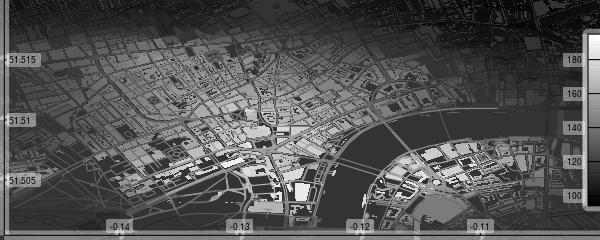R package to produce visually impressive customisable images of OpenStreetMap (OSM) data downloaded internally from the overpass api. The above map was produced directly from `osmplotr` with no further modification. This `README` briefly demonstrates the following functionality:

1. Quick Introduction

2. Installation

3. A Simple Map

4. Highlighting Selected Areas

5. Highlighting Clusters

6. Highlighting Areas Bounded by Named Highways

7. Data Surfaces

8. Gallery

## 1. Quick Introduction

But first the easy steps to map making:

1. Specify the bounding box for the desired region

``bbox <- get_bbox (c(-0.15, 51.5, -0.10, 51.52))``

``dat_B <- extract_osm_objects (key = "building", bbox = bbox)``
3. Initiate an `osm_basemap` with desired background (`bg`) colour

``map <- osm_basemap (bbox = bbox, bg = "gray20")``
4. Overlay objects on plot in the desired colour.

``map <- add_osm_objects (map, dat_B, col = "gray40")``
5. Print the map to graphics device of choice

``print_osm_map (map)``

## 2. Installation

First install the package

``install.packages ("osmplotr")``

or the development version

``devtools::install_github ("ropensci/osmplotr")``

And then load it in the usual way

``library (osmplotr)``

## 3. A Simple Map

Simple maps can be made by overlaying different kinds of OSM data in different colours:

``````dat_H <- extract_osm_objects (key = "highway", bbox = bbox)
dat_P <- extract_osm_objects (key = "park", bbox = bbox)
dat_G <- extract_osm_objects (key = "landuse", value = "grass", bbox = bbox)``````
``````map <- osm_basemap (bbox = bbox, bg = "gray20")
map <- add_osm_objects (map, dat_B, col = "gray40")
map <- add_osm_objects (map, dat_H, col = "gray80")
map <- add_osm_objects (map, dat_P, col = "darkseagreen")
map <- add_osm_objects (map, dat_G, col = "darkseagreen1")
print_osm_map (map)``````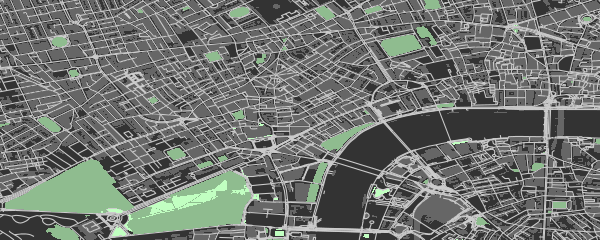## 4. Highlighting Selected Areas

`osmplotr` is primarily intended as a data visualisation tool, particularly through enabling selected regions to be highlighted. Regions can be defined according to simple point boundaries:

``````pts <- sp::SpatialPoints (cbind (c (-0.115, -0.13, -0.13, -0.115),
c (51.505, 51.505, 51.515, 51.515)))``````

OSM objects within the defined regions can then be highlighted with different colour schemes. `cols` defines colours for each group (with only one here), while `bg` defines the colour of the remaining, background area.

``````map <- osm_basemap (bbox = bbox, bg = "gray20")
map <- add_osm_groups (map, dat_B, groups = pts, cols = "orange", bg = "gray40")
map <- add_osm_objects (map, london\$dat_P, col = "darkseagreen1")
map <- add_osm_groups (map, london\$dat_P, groups = pts, cols = "darkseagreen1",
bg = "darkseagreen", boundary = 0)
print_osm_map (map)``````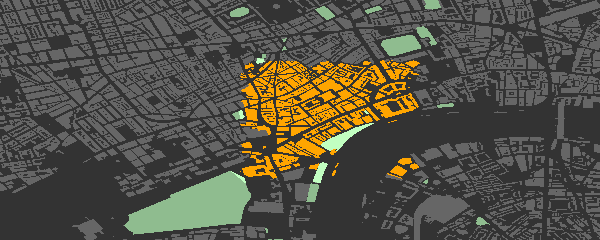Note the `border = 0` argument on the last call divides the park polygons precisely along the border. The same map highlighted in dark-on-light:

``````map <- osm_basemap (bbox = bbox, bg = "gray95")
map <- add_osm_groups (map, dat_B, groups = pts, cols = "gray40", bg = "gray85")
map <- add_osm_groups (map, dat_H, groups = pts, cols = "gray20", bg = "gray70")
print_osm_map (map)``````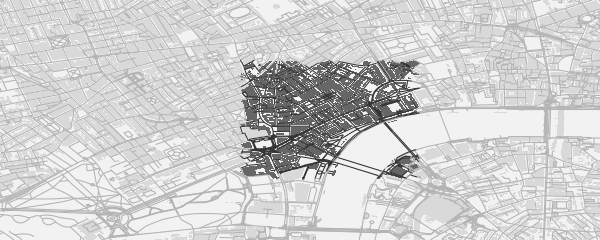## 5. Highlighting Clusters

`add_osm_groups` also enables plotting an entire region as a group of spatially distinct clusters of defined colours. Groups can be defined by simple spatial points denoting their centres:

``````set.seed (2)
ngroups <- 12
x <- bbox [1, 1] + runif (ngroups) * diff (bbox [1, ])
y <- bbox [2, 1] + runif (ngroups) * diff (bbox [2, ])
groups <- cbind (x, y)
groups <- apply (groups, 1, function (i)
sp::SpatialPoints (matrix (i, nrow = 1, ncol = 2)))``````

Calling `add_osm_groups` with no `bg` argument forces all points lying outside those defined groups to be allocated to the nearest groups, and thus produces an inclusive grouping extending across an entire region.

``````map <- osm_basemap (bbox = bbox, bg = "gray20")
map <- add_osm_groups (map, dat_B, groups = groups,
cols = rainbow (length (groups)), border_width = 2)
print_osm_map (map)``````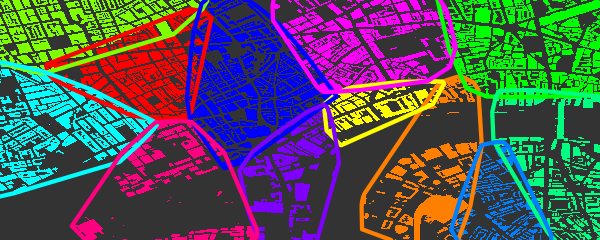## 6. Highlighting Areas Bounded by Named Highways

An alternative way of defining highlighted groups is by naming the highways encircling desired regions.

``````# These highways extend beyond the previous, smaller bbox
bbox_big <- get_bbox (c(-0.15, 51.5, -0.10, 51.52))
highways <- c ("Davies.St", "Berkeley.Sq", "Berkeley.St", "Piccadilly",
"Regent.St", "Oxford.St")
highways1 <- connect_highways (highways = highways, bbox = bbox_big)
highways <- c ("Regent.St", "Oxford.St", "Shaftesbury")
highways2 <- connect_highways (highways = highways, bbox = bbox_big)
highways <- c ("Piccadilly", "Shaftesbury.Ave", "Charing.Cross.R",
"Saint.Martin", "Trafalgar.Sq", "Cockspur.St",
"Pall.Mall", "St.James")
highways3 <- connect_highways (highways = highways, bbox = bbox_big)
highways <- c ("Charing.Cross", "Duncannon.St", "Strand", "Aldwych",
"Kingsway", "High.Holborn", "Shaftesbury.Ave")
highways4 <- connect_highways (highways = highways, bbox = bbox_big)
highways <- c ("Kingsway", "Holborn", "Farringdon.St", "Strand",
"Fleet.St", "Aldwych")
highways5 <- connect_highways (highways = highways, bbox = bbox_big)
groups <- list (highways1, highways2, highways3, highways4, highways5)``````

And then passing these lists of groups returned by `connect_highways` to `add_osm_groups`, this time with some Wes Anderson flair.

``````map <- osm_basemap (bbox = bbox, bg = "gray20")
library (wesanderson)
cols <- wes_palette ("Darjeeling", 5)
map <- add_osm_groups (map, dat_B, groups = groups, boundary = 1,
cols = cols, bg = "gray40", colmat = FALSE)
map <- add_osm_groups (map, dat_H, groups = groups, boundary = 0,
cols = cols, bg = "gray70", colmat = FALSE)
print_osm_map (map)``````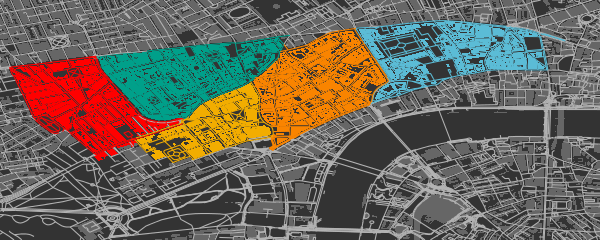## 7. Data Surfaces

Finally, `osmplotr` contains a function `add_osm_surface` that spatially interpolates a given set of spatial data points and colours OSM objects according to a specified colour gradient. This is illustrated here with the `volcano` data projected onto the `bbox`.

``````x <- seq (bbox [1, 1], bbox [1, 2], length.out = dim (volcano))
y <- seq (bbox [2, 1], bbox [2, 2], length.out = dim (volcano))
xy <- cbind (rep (x, dim (volcano) ), rep (y, each = dim (volcano) ))
z <- as.numeric (volcano)
dat <- data.frame (x = xy [, 1], y = xy [, 2], z = z)``````
``````map <- osm_basemap (bbox = bbox, bg = "gray20")
cols <- gray (0:50 / 50)
map <- add_osm_surface (map, dat_B, dat = dat, cols = cols)
# Darken cols by ~20%
map <- add_osm_surface (map, dat_H, dat = dat,